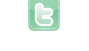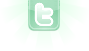## can somebody plz tell what i am missing here

I have this four classes, saving_account, chequing_account, transaction_account and the account class but the question is mostly based on the saving, chequing, account and transaction classes. in all of the classes except for the transaction class(which is pertaining to the transaction class actually) I have this error that says: constructor Transaction_Account in class Transaction_Account cannot be applied to given types; required: Date, char, double, double, String but instead found: found: Date, char, double, int, int, String but when I look in the transaction class, there is date, char, double, double and string. i have check this over a million time and even check all of the other classes and still cant see where the error is originated from. all of the classes have the same error, I am going to post one of the classes with the error and I will also post the transaction class. any help will be appreciated I am posting the saving account class
```public class Saving_Account extends Account{

private  float interestRate = 500;
double balance;

public Saving_Account(String firstname, String acctnum,  double balance, float interestRate){
super(firstname, acctnum, balance);
this.interestRate=interestRate;

}

public float getinterestRate(){
return interestRate;
}

public void setinterestRate(float interestRate){
this.interestRate = interestRate/100;

}

private double getBalance(double balance) {
return balance;
}

public void setbalance(double balance){
this.balance = balance;
}
public void payInterest(){
double total_amt = getbalance();
double balance = 0;
balance=(total_amt + (total_amt*getinterestRate()));
setbalance(balance);

System.out.print("Account Balance with interest is %.2f"+balance);
Transaction_Account newtrans = new Transaction_Account(new Date(), 'I', balance,0,0,"Interest Paid");

}

//withdrawal method
@Override
public void withdraw(double total_amt){
double balance = 0;
double acctamt = 0;
if(acctamt < total_amt){
System.out.println("Insufficient funds, balance is :\$"+ acctamt);
}
else{
balance = acctamt -= total_amt;
setbalance(balance);
System.out.print("The withdrawal of: \$"+total_amt + " was succesful, the new balance is: \$%.2f" + getBalance(balance));
Transaction_Account newtrans = new Transaction_Account(new Date(), 'W', balance, 0,0, "Withdraw Made");
}

}
@Override
public void deposit(double total_amt){
double balance;
double acctamt = getbalance();
balance = acctamt+=total_amt;
setbalance(balance);
System.out.println("Your deposit amount is: \$"+ total_amt + "was successful, the new balance is"+ balance);
Transaction_Account newtrans = new Transaction_Account(new Date(), 'D', balance, 0,0, "deposit made"); this is where the error is:constructor Transaction_Account in class Transaction_Account cannot be applied to given types;
required: Date,char,double,double,String
found: Date,char,double,int,int,String

}
@Override
public String toString(){

return this.toString()+ "Interest Rate"+ interestRate;
}

}

this is the transaction class where it is saying the error is and there is none, it have to correct variable

public class Transaction_Account{

ArrayList <Transaction_Account> newtrans = new ArrayList();

private Date date;
private double balance;
private String description;
private char type;
private double total_amt;

public Transaction_Account(Date date, char type, double balance, double total_amt, String description){

this.date = new Date();
this.type = type;
this.balance = balance;
this.total_amt = total_amt;
this.description=description;

}

public Date getDate(){
return date;
}
public char gettype(){
return type;
}
public double getbalance(){
return balance;
}
public String getdescription(){
return description;
}
public double gettotal_amt(){
}

public void setDate(Date date){
this.date=date;
}
public void settype(char type){
this.type=type;
}
public void setbalance(double balance){
this.balance = balance;
}
public void setdescription(String description){
this.description=description;
}
public void settotal_amt(double total_amt){
this.total_amt = total_amt;
}

@Override
public String toString(){
return "\n"+new Date()+" "+ "Type "+ this.type + " "+ "Account Balance"+ this.balance +" "+"Description"+ this.description;
}

}```
divinity wrote...
when I look in the transaction class, there is date, char, double, double and string.
Yes, but line 36 does not conform to those parameters.
thank you very much danpost found the error